see the demo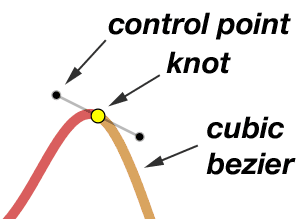# Spline Interpolation

I got into splines the way many people do: I wanted a way to draw smooth, attractive connectors between graphic objects in a very general way, and with the ability to specify the exact path the curves should take. This is what interpolation implies: that the curve will go exactly through the specified points. A little study quickly shows that

1. HTML5 canvas is the only way to go if you're working client-side without plug-ins.
2. The canvas function bezierCurveTo() connects two points with a smooth curve, and so should be able to do most of the work by calling it for each pair of adjacent points we want to connect.
3. Cubic Bezier curves are specified by their endpoints (often called knots) and two control points. But beziers do not interpolate their control points (they do not pass through them), so we can't just pass our list of points to bezierCurveTo(), we are going to have to figure out where those control points need to be. That's what all of this comes down to: where should the control points be for a series of cubic bezier curves, in order to get the appearance we want?
4. The literature on control point location is extensive, often mathematically formal, and excessively complicated. All my intuition says that a simple geometric problem should have a simple geometric solution — and you'll see here that it does.
5. If you don't care about the geometry but just want to get on with it, my code (the source code for the demo) draws nice connected curves (i.e. interpolating splines) with one call: drawSpline(ctx, points, t, closedCurve), where ctx is the canvas context object, points is a simple array [x0,y0,x1,y1,...xn,yn] of the points we want to connect, t is a constant, usually 0.3 to 0.5, which controls the smoothness according to your taste, and closedCurve is a boolean to say whether or not the endpoints should be smoothly connected. The animation in the demo slides t from 0.5 to 1 to 0 to 0.333, to give you a feel for what that implies.

## Requirements

The curved segments (= splines) must go through every knot point. They must connect at the knots "smoothly", that is, have the same slope (first derivative) there. They need not have the same curvature (second derivative) at the knots; that's desirable but there are only so many degrees of freedom in a cubic polynomial — can't have it all. We should be able to tweak the smoothness of the curves: for example if we're doing strict rectangular layouts (like city blocks), we may want fairly tight curves at the knots. We should be able, optionally, to connect the ends of the curve and have the whole closed curve be smooth (no cusps). The implementation should be compact, stable, fast, and easy to drop into any canvas-enabled Javascript page.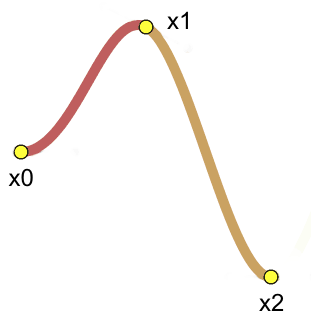Figure 1: Our goal: two cubic bezier curves connecting at x1.

## The Geometry

Figure 1 shows the goal: if we can create two bezier curves (shown in red and orange) that begin and end at points x0 and x2 and connect smoothly at point x1, then we can repeat the process and splice together any number of knot points.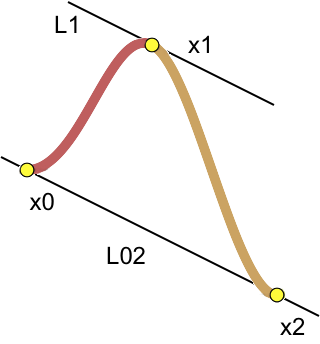Figure 2: Baselines

The key learnings from the literature on bezier splines are first, that for a smooth connection the control points flanking a knot must lie on a line that passes through the knot, and second, that line should be parallel to a line connecting the flanking knots. This shown in Figure 2, where our control points will lie somewhere on line L1, which is parallel to line L02.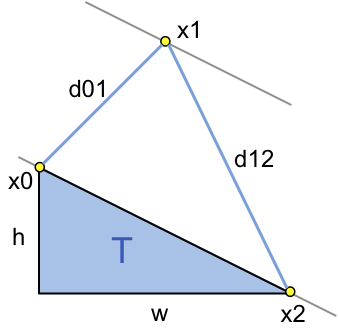Figure 3: Parent triangle and inter-knot distances

With some erasure to make the sketch clearer, Figure 3 shows more things we need: first, the distances between the knots, d01 and d12. Those are very easily calculated from the knot (x,y) coordinates which are our input data. Also we need right triangle T, which connects x0 and x2 and has width w and height h, also easily calculated from the knot coordinates.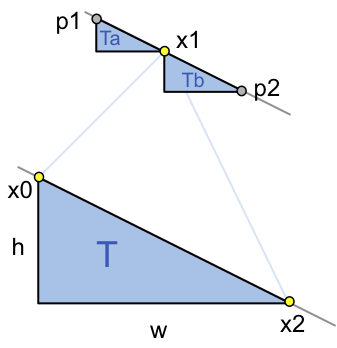Figure 4: Similar triangles define the control points

The last step is to draw two smaller triangles, similar to T (same angles and orientation), with their long edges along line L1 (they're parallel) and connecting at point x1. These smaller triangles Ta and Tb are scaled down from T by two factors: first, the distances d01 and d12, respectively, and secondly by the constant parameter t. Scaling proportionate to inter-knot distance is a simple way to get appropriate curvature: if two knots are very close, then the control points between them should be close to the knots to assure a "tight corner". The scaling of T gives us the widths and heights of Ta and Tb, and with those the coordinates of control points p1 and p2 are easily found from point x1. Here's the Javascript:

```function getControlPoints(x0,y0,x1,y1,x2,y2,t){
var d01=Math.sqrt(Math.pow(x1-x0,2)+Math.pow(y1-y0,2));
var d12=Math.sqrt(Math.pow(x2-x1,2)+Math.pow(y2-y1,2));
var fa=t*d01/(d01+d12);   // scaling factor for triangle Ta
var fb=t*d12/(d01+d12);   // ditto for Tb, simplifies to fb=t-fa
var p1x=x1-fa*(x2-x0);    // x2-x0 is the width of triangle T
var p1y=y1-fa*(y2-y0);    // y2-y0 is the height of T
var p2x=x1+fb*(x2-x0);
var p2y=y1+fb*(y2-y0);
return [p1x,p1y,p2x,p2y];
}
```

Everything else in the code is just bookkeeping. In these sketches we found two control points, but for different bezier curves: control point p1 (Figure 4) is needed to draw the left bezier (red in Figures 1 & 2) and p2 is needed to draw the right (orange) bezier. This just means that we have to calculate all of the control points (or at least those a knot fore and aft of where we are) before drawing. Closed curves need the control points at the "beginning" and "end" points (wherever you start and end), more bookkeeping. But the result is a simple, fast bezier spline routine with only one parameter to adjust the curvature.

Note in the demo that when t=0 the curves become straight lines connecting the knot points, and when t=1 the curves are "too curvy" for the open zigzag curve, but actually for the square (lower left), t=1 makes a nice "rounded square" that might be a useful shape. There is no upper bound to t, but above t=1 you're almost guaranteed to get distracting cusps and loops. For that matter, t can be negative, which is great for drawing knots.

Rob Spencer, July 2010